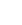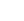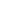From experimental approximation to the order of magnitude of theoretical probability

# From experimental approximation to the order of magnitude of theoretical probability

There are problems where it is impossible to determine the theoretical probability of an event and is only possible through a huge number of simulations to obtain an experimental one. In this project we try to find a mathematical relationship between the magnitude of the theoretical probability of an event and the number of Monte Carlo simulations needed to obtain a close experimental probability.
The investigation was carried out by doing simulations of tosses of various dice (2 to 5), and analyzing the behavior of the experimental probability, varying the number of simulations of throws (up to one thousand million), to verify in how many simulations the experimental probability of rolling out 1 on all dice is within a 5% deviation from the theoretical one, for each number of dice.

19 years

19 years

### Share on Social Media## Bezoekers / Local Visitors## FAQ## European Year of Youth## Contact